# Magnetic- and Electric- field lines due to a moving magnetic monopole

milkism
Homework Statement:
Find the magnetic- and field lines equations of a moving magnetic monopole charge at constant velocirty v.
Relevant Equations:
#
Question: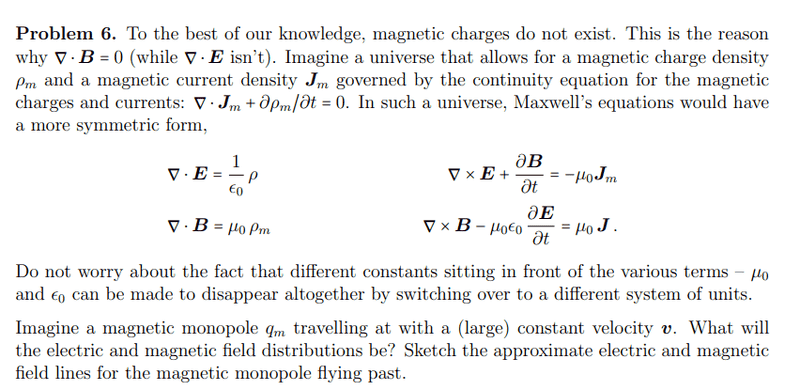What it looks like for an electric charge: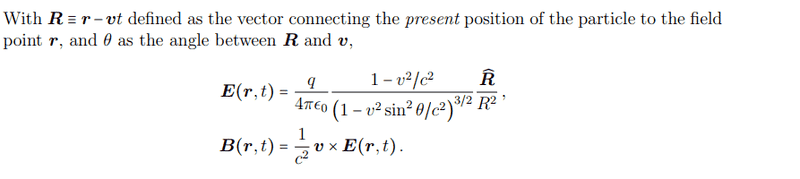Am I correct? If you want I can hand out my Latex on how I got to it, it will refer to the book Griffiths a lot.

Homework Helper
Gold Member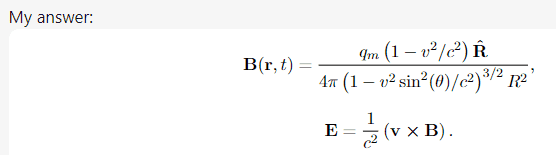You didn't show how you got these results. You can use dimensional analysis to see that these aren't correct.

•milkism and jim mcnamara
milkism
You didn't show how you got these results. You can use dimensional analysis to see that these aren't correct.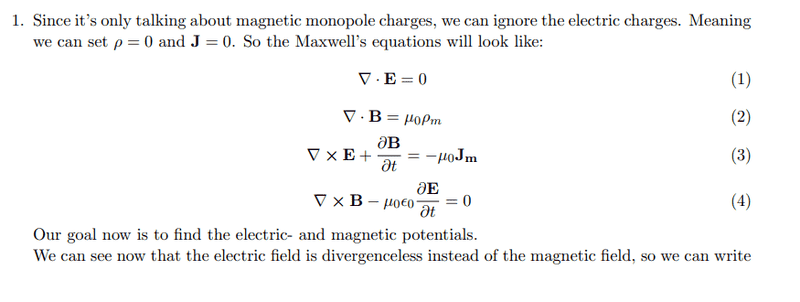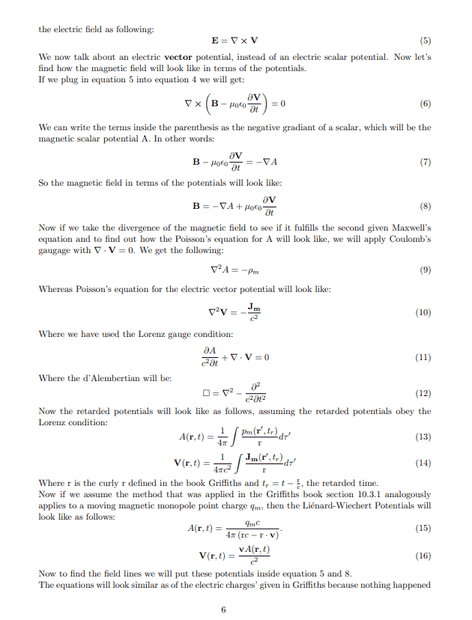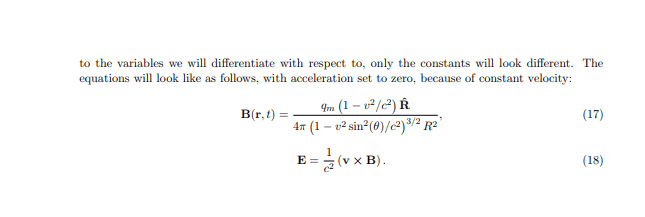I think my retarded potentials are wrong, because of wrong constants should for A(r,t) there to be a mu_0 and for V(r,t) instead of 1/c², an 1/epsilon_zero?

Homework Helper
Gold Member
I haven't checked the details of your calculation using the approach of rederiving scalar and vector potentials for the fields due to magnetic charge and then using these potentials to derive the fields of a uniformly moving magnetic point charge (monopole). It looks tedious.

Another approach is to compare Maxwell's equations for electric charge with Maxwell's equations for magnetic charge.

For electric charge (with no magnetic charge present) we have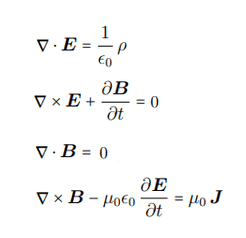For magnetic charge (with no electric charge present) we have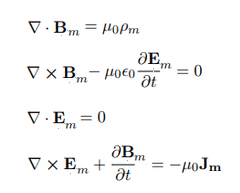For convenience, I've added the subscript ##m## for the fields due to magnetic charge.

These two sets of equations are equivalent except for symbols. For example, you can convert the first equation in the electric charge equations to the first equation in the magnetic equations by making the symbolic substitutions $$\mathbf{E} \rightarrow \mathbf{B_m}$$ $$\rho \rightarrow \mu_0 \epsilon_0 \rho_m$$

See if you can find additional substitutions $$\mathbf{B} \rightarrow [?]$$ $$\mathbf{J} \rightarrow [?]$$ so that the other equations for the electric charge are transformed into the other equations for the magnetic charge.

You can then use these substitutions to directly convert the expressions for the fields of the uniformly moving electric charge into the expressions for the fields of the uniformly moving magnetic charge.

•MatinSAR, milkism and vanhees71
Gold Member
2022 Award
BTW: That's known as the "duality transformation". Including magnetic charge in Maxwell's equations makes them entirely invariant under such transformations, and indeed you can use this to get the result from the known field of a moving electric charge.

Another simple way is to calculate the solution for a magnetic charge at rest and then Lorentz boost the fields. That's technically simpler than using the Lienard-Wiechert (retarded) potentials.

•MatinSAR, milkism and TSny
milkism
I haven't checked the details of your calculation using the approach of rederiving scalar and vector potentials for the fields due to magnetic charge and then using these potentials to derive the fields of a uniformly moving magnetic point charge (monopole). It looks tedious.

Another approach is to compare Maxwell's equations for electric charge with Maxwell's equations for magnetic charge.

For electric charge (with no magnetic charge present) we have
View attachment 323535

For magnetic charge (with no electric charge present) we have

View attachment 323536
For convenience, I've added the subscript ##m## for the fields due to magnetic charge.

These two sets of equations are equivalent except for symbols. For example, you can convert the first equation in the electric charge equations to the first equation in the magnetic equations by making the symbolic substitutions $$\mathbf{E} \rightarrow \mathbf{B_m}$$ $$\rho \rightarrow \mu_0 \epsilon_0 \rho_m$$

See if you can find additional substitutions $$\mathbf{B} \rightarrow [?]$$ $$\mathbf{J} \rightarrow [?]$$ so that the other equations for the electric charge are transformed into the other equations for the magnetic charge.

You can then use these substitutions to directly convert the expressions for the fields of the uniformly moving electric charge into the expressions for the fields of the uniformly moving magnetic charge.
Is it B to (E_m)/(y_0 e_0) and J to -J_m?

Homework Helper
Gold Member
Is it B to (E_m)/(y_0 e_0) and J to -J_m?
No, neither of these is correct.

For example, start with the Maxwell equation $$\nabla \times \mathbf{E} + \frac{\partial \mathbf{B}}{\partial t} = 0.$$ In post #4 we have ##\mathbf{E} \rightarrow \mathbf{B_m}##. Suppose we also assume your correspondence ##\mathbf{B} \rightarrow \mathbf{E_m}/(\mu_0 \epsilon_0)##. Then this Maxwell equation becomes $$\nabla \times \mathbf{B_m} +\frac 1 {\mu_0 \epsilon_0} \frac{\partial \mathbf{E_m}}{\partial t} = 0.$$ But the equation we need to obtain is $$\nabla \times \mathbf{B_m} -\mu_0 \epsilon_0 \frac{\partial \mathbf{E_m}}{\partial t} = 0.$$

•MatinSAR and milkism
milkism
No, neither of these is correct.

For example, start with the Maxwell equation $$\nabla \times \mathbf{E} + \frac{\partial \mathbf{B}}{\partial t} = 0.$$ In post #4 we have ##\mathbf{E} \rightarrow \mathbf{B_m}##. Suppose we also assume your correspondence ##\mathbf{B} \rightarrow \mathbf{E_m}/(\mu_0 \epsilon_0)##. Then this Maxwell equation becomes $$\nabla \times \mathbf{B_m} +\frac 1 {\mu_0 \epsilon_0} \frac{\partial \mathbf{E_m}}{\partial t} = 0.$$ But the equation we need to obtain is $$\nabla \times \mathbf{B_m} -\mu_0 \epsilon_0 \frac{\partial \mathbf{E_m}}{\partial t} = 0.$$
$$\mathbf{B} \rightarrow -(\mu_0 \epsilon_0 )\mathbf{E_m}$$, and for last one $$\mathbf{E} \rightarrow - \frac{ \mathbf{B_m}}{\mu_0 \epsilon_0}, \mathbf{J} \rightarrow - \mathbf{J_m}$$?

Homework Helper
Gold Member
$$\mathbf{B} \rightarrow -(\mu_0 \epsilon_0 )\mathbf{E_m}$$
Yes, this looks good.

and for last one $$\mathbf{E} \rightarrow - \frac{ \mathbf{B_m}}{\mu_0 \epsilon_0}, \mathbf{J} \rightarrow - \mathbf{J_m}$$
No. From post #4, we started with the transcriptions ##\mathbf{E} \rightarrow \mathbf{B_m}## and ##\mathbf{\rho} \rightarrow \mu_0 \epsilon_0 \rho_m##. So, we must retain these as we figure out the other transcriptions. So, at this point, you have $$\mathbf{E} \rightarrow \mathbf{B_m} , \,\,\,\,\, \mathbf{\rho} \rightarrow \mu_0 \epsilon_0 \rho_m \,\,\,\,\, \rm{and} \,\,\,\,\ \mathbf{B} \rightarrow -\mu_0\epsilon_0 \mathbf{E_m}$$ You just need to get the correct relation between the symbols ##\mathbf{J}## and ##\mathbf{J_m}##.

[Note: We could have started out in post #4 with the different choice ##\mathbf{E} \rightarrow- \mathbf{B_m}## and ##\mathbf{\rho} \rightarrow -\mu_0 \epsilon_0 \rho_m## which has negative signs on the right sides. This choice would still make ##\nabla \cdot \mathbf{E} = \rho/\epsilon_0## transcsribe to the equation ##\nabla \cdot \mathbf{B_m} = \mu_0 \rho_m## , as required. Then the transcriptions for the other symbols would need to be modified appropriately. So, the transcription that will map the "electric charge" Maxwell equations to the "magnetic charge" Maxwell equations is not unique. (The number of choices is infinite.) But these different choices would all lead to the same result for the fields of the uniformly moving magnetic monopole. So, you just need to find any one particular set of transcriptions of the symbols that will work.]

•MatinSAR and milkism
milkism
Yes, this looks good.

No. From post #4, we started with the transcriptions ##\mathbf{E} \rightarrow \mathbf{B_m}## and ##\mathbf{\rho} \rightarrow \mu_0 \epsilon_0 \rho_m##. So, we must retain these as we figure out the other transcriptions. So, at this point, you have $$\mathbf{E} \rightarrow \mathbf{B_m} , \,\,\,\,\, \mathbf{\rho} \rightarrow \mu_0 \epsilon_0 \rho_m \,\,\,\,\, \rm{and} \,\,\,\,\ \mathbf{B} \rightarrow -\mu_0\epsilon_0 \mathbf{E_m}$$ You just need to get the correct relation between the symbols ##\mathbf{J}## and ##\mathbf{J_m}##.

[Note: We could have started out in post #4 with the different choice ##\mathbf{E} \rightarrow- \mathbf{B_m}## and ##\mathbf{\rho} \rightarrow -\mu_0 \epsilon_0 \rho_m## which has negative signs on the right sides. This choice would still make ##\nabla \cdot \mathbf{E} = \rho/\epsilon_0## transcsribe to the equation ##\nabla \cdot \mathbf{B_m} = \mu_0 \rho_m## , as required. Then the transcriptions for the other symbols would need to be modified appropriately. So, the transcription that will map the "electric charge" Maxwell equations to the "magnetic charge" Maxwell equations is not unique. (The number of choices is infinite.) But these different choices would all lead to the same result for the fields of the uniformly moving magnetic monopole. So, you just need to find any one particular set of transcriptions of the symbols that will work.]
$$\mathbf{J} \rightarrow -\mathbf{J_m}\left(\mu _0 \epsilon _0\right)$$?

Last edited:
Homework Helper
Gold Member
$$\mathbf{J} \rightarrow -\mathbf{J_m}\left(\mu _0 \epsilon _0\right)$$
That's very close. Please show the steps that you used to get this.

•milkism
Gold Member
2022 Award
Just look at the (microscopic) Maxwell equations (in SI units) including magnetic monopoles
$$\vec{\nabla} \cdot \vec{E}=\frac{1}{\epsilon_0} \rho_{\text{el}}, \quad \vec{\nabla} \cdot \vec{B}=\rho_{\text{mag}},$$
$$\vec{\nabla} \times \vec{B}-\epsilon_0 \mu_0 \partial_t \vec{E} = \mu_0 \vec{j}, \quad -\partial_t \vec{B}-\vec{\nabla} \times \vec{E}=\vec{j}_{\text{mag}}.$$
Now think about how to exchange ##\vec{E}## and ##\vec{B}## simultaneously with ##\rho_{\text{el}}## and ##\rho_{\text{mag}}## and ##\vec{j}_{\text{el}}## with ##\vec{j}_{\text{mag}}## with appropriate factors and signs such that the equations don't change. That are the celebrated "duality transformations".

Note that everything gets much simpler when you use Gaussian or Heaviside-Lorentz units ;-). Also the covariant relativistic formulation is revealing!

•milkism
milkism
That's very close. Please show the steps that you used to get this.
$$-\left( \mu_0 \epsilon_0 \right) \nabla \cross \mathbf{E_m} - \left( \mu_0 \epsilon_0 \right) \frac{\partial \mathbf{B_m}{\partial t} = \mu_0 \mathbf{J} \left(-\mu_0 \epsilon_0 \right) \left( \nabla \cross \mathbf{E_m} + \frac{\partial \mathbf{B_m}{\partial t} \right)= \mu_0 \mathbf{J} \nabla \cross \mathbf{E_m} + \frac{\partial \mathbf{B_m}{\partial} = \frac{\mu_0 \mathbf{J}}{- \mu_0 \epsilon _0}$$So $$\mathbf{J} \rightarrow -\left( \mu_0 \epsilon_0 \right) \mathbf{J_m}$$. (No idea why Latex doesn't work)

Homework Helper
Gold Member
$$-\left( \mu_0 \epsilon_0 \right) \nabla \times \mathbf{E_m} - \left( \mu_0 \epsilon_0 \right) \frac{\partial \mathbf{B_m}}{\partial t} = \mu_0 \mathbf{J}$$ $$\left(-\mu_0 \epsilon_0 \right) \left( \nabla \times \mathbf{E_m} + \frac{\partial \mathbf{B_m}}{\partial t} \right)= \mu_0 \mathbf{J}$$ $$\nabla \times \mathbf{E_m} + \frac{\partial \mathbf{B_m}}{\partial t} = \frac{\mu_0 \mathbf{J}}{- \mu_0 \epsilon _0}$$
So $$\mathbf{J} \rightarrow -\left( \mu_0 \epsilon_0 \right) \mathbf{J_m}$$.
There were some missing } symbols in the formatting and I added some $symbols to make the individual equations show on separate lines. Your work looks correct except for the final conclusion. You ended up with the equation $$\nabla \times \mathbf{E_m} + \frac{\partial \mathbf{B_m}}{\partial t} = \frac{\mu_0 \mathbf{J}}{- \mu_0 \epsilon _0}$$ that you want to make equivalent to the equation $$\nabla \times \mathbf{E_m} + \frac{\partial \mathbf{B_m}}{\partial t} = -\mu_0 \mathbf{J_m}$$ Note that both equations have a negative sign on the right side. •milkism milkism There were some missing } symbols in the formatting and I added some$ symbols to make the individual equations show on separate lines. Your work looks correct except for the final conclusion. You ended up with the equation $$\nabla \times \mathbf{E_m} + \frac{\partial \mathbf{B_m}}{\partial t} = \frac{\mu_0 \mathbf{J}}{- \mu_0 \epsilon _0}$$ that you want to make equivalent to the equation $$\nabla \times \mathbf{E_m} + \frac{\partial \mathbf{B_m}}{\partial t} = -\mu_0 \mathbf{J_m}$$ Note that both equations have a negative sign on the right side.
So it's just positive. So electric- and magnetic field formulae due to electric charge are (ignoring the other constants and distance)
$$\mathbf{E} = \frac{\rho \tau}{\epsilon_0}$$
$$\mathbf{B} = \mu_0 \mathbf{J} \tau$$
For magnetic monopoles it will become
$$\mathbf{B_m} = \mu_0 \rho _m \tau$$
$$\mathbf{E_m} = \mu_0 \mathbf{J_m} \tau$$
? Where tau is volume.

Homework Helper
Gold Member
So it's just positive. So electric- and magnetic field formulae due to electric charge are (ignoring the other constants and distance)
$$\mathbf{E} = \frac{\rho \tau}{\epsilon_0}$$
$$\mathbf{B} = \mu_0 \mathbf{J} \tau$$
For magnetic monopoles it will become
$$\mathbf{B_m} = \mu_0 \rho _m \tau$$
$$\mathbf{E_m} = \mu_0 \mathbf{J_m} \tau$$
? Where tau is volume.
I don't think you took into account the negative sign in the relation ##\mathbf{B} \rightarrow -\mu_0 \epsilon_0 \mathbf{E}_m##.

Also, I'm guessing that you've somehow introduced the volume of the charge, ##\tau##, with the intention of replacing ##\rho \tau## by ##q##.

However, it's not so simple. For example, the potentials ##V## and ##\mathbf{A}## depend on the sources ##\rho## and ##\mathbf{J}## at retarded times ##t_r##. This is discussed in Griffiths (pages 430 - 431 in the 3rd edition) or see section 21-5 of the Feynman Lectures. As shown there, it turns out that ##\int \rho(\mathbf{r'}, t_r) d\tau' \neq q##.

But, we don't need to worry about this because exactly the same subtleties occur for the magnetic monopole. The transcription ##\rho \rightarrow \mu_0 \epsilon_0 \rho_m## means that ##q## will get replaced by ##\mu_0 \epsilon_0 q_m## in the final equations.

So, the formula for the electric field of a moving electric charge is given to be $$\mathbf{E}(r, t) = \frac{q}{4 \pi \epsilon_0}\frac{1-v^2/c^2}{\left(1-v^2 \sin^2 \theta /c^2 \right)^{3/2}}\frac{\hat{\mathbf{R}}}{R^2}$$ It should now be easy to translate this to the expression for ##\mathbf{B}_m(r, t)## for the magnetic monopole.

Likewise, you can translate ##\mathbf{B}(\mathbf{r}, t) = \frac 1 {c^2} \mathbf{v} \times \mathbf{E}(\mathbf{r},t)## to get ##\mathbf{E}_m(\mathbf{r},t)##.

Last edited:
•milkism
milkism
I don't think you took into account the negative sign in the relation ##\mathbf{B} \rightarrow -\mu_0 \epsilon_0 \mathbf{E}_m##.

Also, I'm guessing that you've somehow introduced the volume of the charge, ##\tau##, with the intention of replacing ##\rho \tau## by ##q##.

However, it's not so simple. For example, the potentials ##V## and ##\mathbf{A}## depend on the sources ##\rho## and ##\mathbf{J}## at retarded times ##t_r##. This is discussed in Griffiths (pages 430 - 431 in the 3rd edition) or see section 21-5 of the Feynman Lectures. As shown there, it turns out that ##\int \rho(\mathbf{r'}, t_r) d\tau' \neq q##.

But, we don't need to worry about this because exactly the same subtleties occur for the magnetic monopole. The transcription ##\rho \rightarrow \mu_0 \epsilon_0 \rho_m## means that ##q## will get replaced by ##\mu_0 \epsilon_0 q_m## in the final equations.

So, the formula for the electric field of a moving electric charge is given to be $$\mathbf{E}(r, t) = \frac{q}{4 \pi \epsilon_0}\frac{1-v^2/c^2}{\left(1-v^2 \sin^2 \theta /c^2 \right)^{3/2}}\frac{\hat{\mathbf{R}}}{R^2}$$ It should now be easy to translate this to the expression for ##\mathbf{B}_m(r, t)## for the magnetic monopole.

Likewise, you can translate ##\mathbf{B}(\mathbf{r}, t) = \frac 1 {c^2} \mathbf{v} \times \mathbf{E}(\mathbf{r},t)## to get ##\mathbf{E}_m(\mathbf{r},t)##.
$$\mathbf{B}(\mathbf{r},t) = \frac{q_m \mu _0 \left(1 - v^2 / c^2 \right) \mathbf{\hat{R}}}{4 \pi \left( 1 - v^2 \sin^2 (\theta )/c ^2 \right)^{3/2}R^2},$$
$$\mathbf{E}(\mathbf{r},t) = -\frac{1}{c^4} \left( \mathbf{v} \times \mathbf{B}(\mathbf{r},t) \right).$$

Homework Helper
Gold Member
$$\mathbf{B}(\mathbf{r},t) = \frac{q_m \mu _0 \left(1 - v^2 / c^2 \right) \mathbf{\hat{R}}}{4 \pi \left( 1 - v^2 \sin^2 (\theta )/c ^2 \right)^{3/2}R^2},$$
This looks right.

$$\mathbf{E}(\mathbf{r},t) = -\frac{1}{c^4} \left( \mathbf{v} \times \mathbf{B}(\mathbf{r},t) \right).$$
The factor of ##\large \frac 1 {c^4}## is not correct. If you show your steps, we can find where you made a mistake.

•MatinSAR, vanhees71 and milkism
milkism
This looks right.

The factor of ##\large \frac 1 {c^4}## is not correct. If you show your steps, we can find where you made a mistake.
Originally it was $$\frac{1}{c^2}$$ but because of the $$-c^{2} \mathbf{E}$$ transformation it became $$-\frac{1}{c^4}$$.

Homework Helper
Gold Member
but because of the $$-c^{2} \mathbf{E}$$ transformation it became $$-\frac{1}{c^4}$$.
The transforms are ##\mathbf{E} \rightarrow \mathbf{B}_m## and ##\mathbf{B} \rightarrow -\mu_0 \epsilon_0\mathbf{E}_m##

Note that the second one can be written as ##\mathbf{B} \rightarrow -\mathbf{E}_m/c^2 ##.

So, when translating ##\mathbf{B}(\mathbf{r}, t) = \frac 1 {c^2} \mathbf{v} \times \mathbf{E}(\mathbf{r},t)##, I don't see how you get a factor of ##\large \frac 1 {c^4}##.

•MatinSAR and milkism
milkism
The transforms are ##\mathbf{E} \rightarrow \mathbf{B}_m## and ##\mathbf{B} \rightarrow -\mu_0 \epsilon_0\mathbf{E}_m##

Note that the second one can be written as ##\mathbf{B} \rightarrow -\mathbf{E}_m/c^2 ##.

So, when translating ##\mathbf{B}(\mathbf{r}, t) = \frac 1 {c^2} \mathbf{v} \times \mathbf{E}(\mathbf{r},t)##, I don't see how you get a factor of ##\large \frac 1 {c^4}##.
Oh I misread the formula I thought c² was y_o*e_o, so it basically becomes -v x B.

•vanhees71
Homework Helper
Gold Member
Oh I misread the formula I thought c² was y_o*e_o, so it basically becomes -v x B.
Yes, that's right. Good.

•MatinSAR, vanhees71 and milkism
Homework Helper
Gold Member
When you sketch the E-field lines of the moving magnetic monopole, be sure to take into account the negative sign in ##\mathbf{E}_m = -\mathbf{v} \times \mathbf{B}_m##.

•MatinSAR and milkism
milkism
When you sketch the E-field lines of the moving magnetic monopole, be sure to take into account the negative sign in ##\mathbf{E}_m = -\mathbf{v} \times \mathbf{B}_m##.
Yep, thanks for everything! :D

•TSny